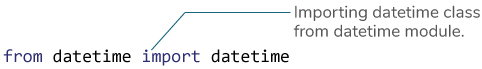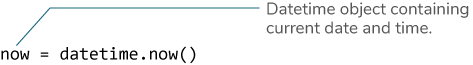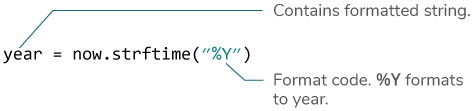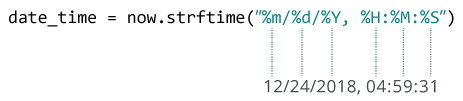# Python strftime()

In this article, you will learn to convert date, time and datetime objects to its equivalent string (with the help of examples)

The `strftime()` method returns a string representing date and time using date, time or datetime object.

## Example 1: datetime to string using strftime()

The program below converts a `datetime` object containing current date and time to different string formats.

`​from datetime import datetime​now = datetime.now() # current date and time​year = now.strftime("%Y")print("year:", year)​month = now.strftime("%m")print("month:", month)​day = now.strftime("%d")print("day:", day)​time = now.strftime("%H:%M:%S")print("time:", time)​date_time = now.strftime("%m/%d/%Y, %H:%M:%S")print("date and time:",date_time)	`

When you run the program, the output will something like be:

`​year: 2018month: 12day: 24time: 04:59:31date and time: 12/24/2018, 04:59:31`

Here, year, day, time and date_time are strings, whereas now is a `datetime` object.

## How strftime() works?

In the above program, `%Y`, `%m`, `%d` etc. are format codes. The `strftime()` method takes one or more format codes as an argument and returns a formatted string based on it.

1. We imported `datetime` class from the `datetime` module. It's because the object of `datetime` class can access `strftime()` method.2. The `datetime` object containing current date and time is stored in now variable.3. The `strftime()` method can be used to create formatted strings.4. The string you pass to the `strftime()` method may contain more than one format codes.## Example 2: Creating string from a timestamp

`​from datetime import datetime​timestamp = 1528797322date_time = datetime.fromtimestamp(timestamp)​print("Date time object:", date_time)​d = date_time.strftime("%m/%d/%Y, %H:%M:%S")print("Output 2:", d)	​d = date_time.strftime("%d %b, %Y")print("Output 3:", d)​d = date_time.strftime("%d %B, %Y")print("Output 4:", d)​d = date_time.strftime("%I%p")print("Output 5:", d)`

When you run the program, the output will be:

`Date time object: 2018-06-12 09:55:22Output 2: 06/12/2018, 09:55:22Output 3: 12 Jun, 2018Output 4: 12 June, 2018Output 5: 09AM`

## Format Code List

The table below shows all the codes that you can pass to the `strftime()` method.

 Directive Meaning Example `%a` Abbreviated weekday name. Sun, Mon, ... `%A` Full weekday name. Sunday, Monday, ... `%w` Weekday as a decimal number. 0, 1, ..., 6 `%d` Day of the month as a zero-padded decimal. 01, 02, ..., 31 `%-d` Day of the month as a decimal number. 1, 2, ..., 30 `%b` Abbreviated month name. Jan, Feb, ..., Dec `%B` Full month name. January, February, ... `%m` Month as a zero-padded decimal number. 01, 02, ..., 12 `%-m` Month as a decimal number. 1, 2, ..., 12 `%y` Year without century as a zero-padded decimal number. 00, 01, ..., 99 `%-y` Year without century as a decimal number. 0, 1, ..., 99 `%Y` Year with century as a decimal number. 2013, 2019 etc. `%H` Hour (24-hour clock) as a zero-padded decimal number. 00, 01, ..., 23 `%-H` Hour (24-hour clock) as a decimal number. 0, 1, ..., 23 `%I` Hour (12-hour clock) as a zero-padded decimal number. 01, 02, ..., 12 `%-I` Hour (12-hour clock) as a decimal number. 1, 2, ... 12 `%p` Locale’s AM or PM. AM, PM `%M` Minute as a zero-padded decimal number. 00, 01, ..., 59 `%-M` Minute as a decimal number. 0, 1, ..., 59 `%S` Second as a zero-padded decimal number. 00, 01, ..., 59 `%-S` Second as a decimal number. 0, 1, ..., 59 `%f` Microsecond as a decimal number, zero-padded on the left. 000000 - 999999 `%z` UTC offset in the form +HHMM or -HHMM. ​ `%Z` Time zone name. ​ `%j` Day of the year as a zero-padded decimal number. 001, 002, ..., 366 `%-j` Day of the year as a decimal number. 1, 2, ..., 366 `%U` Week number of the year (Sunday as the first day of the week). All days in a new year preceding the first Sunday are considered to be in week 0. 00, 01, ..., 53 `%W` Week number of the year (Monday as the first day of the week). All days in a new year preceding the first Monday are considered to be in week 0. 00, 01, ..., 53 `%c` Locale’s appropriate date and time representation. Mon Sep 30 07:06:05 2013 `%x` Locale’s appropriate date representation. 09/30/13 `%X` Locale’s appropriate time representation. 07:06:05 `%%` A literal '%' character. %

## Example 3: Locale's appropriate date and time

`​from datetime import datetime​timestamp = 1528797322date_time = datetime.fromtimestamp(timestamp)​d = date_time.strftime("%c")print("Output 1:", d)	​d = date_time.strftime("%x")print("Output 2:", d)​d = date_time.strftime("%X")print("Output 3:", d)`

When you run the program, the output will be:

`​Output 1: Tue Jun 12 09:55:22 2018Output 2: 06/12/18Output 3: 09:55:22`

Format codes `%c`, `%x` and `%X` are used for locale's appropriate date and time representation.

We also recommend you to check Python strptime(). The `strptime()` method creates a `datetime` object from a string.